### Day 07 - Infinite Limits - 08.25.15

Update
• N/A

Bell Ringer
1. Find the following limit: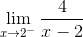1.2.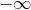3. 0

4. 4

2. Find the following limit: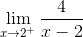1.2.3. 0

4. 4

3. Find the following limit: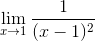1.2.3. 0

4. 1

4. Find the following limit: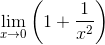1.2.3. 0

4. 1

5. Write your favorite song and its artist. Use the "Other" text box.

Review
• Math Overview (video)
• Numbers
• Relationships
• Shapes
• Change
• Limits
• Intro to Limits (video)
• Nonexistent Limits (video)
• How are limits found numerically and graphically? (checkpoints)
• How are limits found algebraically? (checkpoints)
• Continuity (videoand One-sided Limits (video)
• How can discontinuity of a function be described?
• How are one-sided limits related to regular limits? (checkpoints)

Lesson
• Challenge 4
• Create a function that has an asymptote at x = -3 and a hole at x = 7.

Exit Ticket
• Posted on the board at the end of the block

Homework
Lesson Objectives
• How are infinite limits evaluated?

#### In-Class Help Requests

Standard(s)
• APC.2
• Define and apply the properties of limits of functions.
• Limits will be evaluated graphically and algebraically.
• Includes:
• ​limits of a constant
• ​limits of a sum, product, and quotient
• ​one-sided limits
• ​limits at infinity, infinite limits, and non-existent limits*
• APC.3
• Use limits to define continuity and determine where a function is continuous or discontinuous.
• Includes:
• ​continuity in terms of limits
• continuity at a point and over a closed interval
• ​application of the Intermediate Value Theorem and the Extreme Value Theorem
• ​geometric understanding and interpretation of continuity and discontinuity